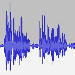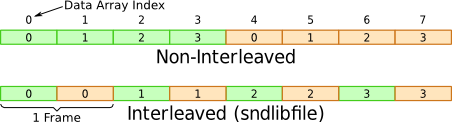# The Lab Book Pages

An online collection of electronics information

Dr. Andrew Greensted# Wav Files

This page contains details on handling Wav files. On the whole these store audio data, but there is no reason why they can't be used for storing any stream data, such as data from an oscilloscope.

## Reading and Writing Wav Files Using Java

Reading and writing wav files using the standard Java API is possible, but not particularly easy. Therefore, I've written an easy to use class for this purpose. Full details with examples and documentation can be found on the Java Wav File IO page.

## Reading and Writing Wav Files Using C

The `libsndfile` library provides a very simple method for dealing with wav files in c. Note: Although we are dealing with wav files, the `libsndfile` library can deal with many more file formats.

The following example shows how to read in a wav file. In this case, the programme is expecting a single channel (mono), 16bit PCM encoded file. The complete source file can be downloaded using the link below.

`readWav.c`

It is compiled and executed as follows:

```> gcc -lsndfile -o readWav readWav.c
```

A description of the programme now follows.

The important include here is `sndfile.h`, this provides access to the `libsndfile` library functions. `malloc.h` is used for creating storage for the loaded wav file.

```#include <stdio.h>
#include <malloc.h>
#include <sndfile.h>
```

Just a basic check that the command line argument for the wav file to be loaded has been given.

```int main(int argc, char *argv[])
{
if (argc != 2) {
fprintf(stderr, "Expecting wav file as argument\n");
return 1;
}
```

This opens the wav file and files the `sndInfo` structure with information about the file.

```   // Open sound file
SF_INFO sndInfo;
SNDFILE *sndFile = sf_open(argv, SFM_READ, &sndInfo);
if (sndFile == NULL) {
fprintf(stderr, "Error reading source file '%s': %s\n", argv, sf_strerror(sndFile));
return 1;
}
```

We now check the format of the file we are reading in. This isn't strictly necessary, but it's here just to demonstrate.

```   // Check format - 16bit PCM
if (sndInfo.format != (SF_FORMAT_WAV | SF_FORMAT_PCM_16)) {
fprintf(stderr, "Input should be 16bit Wav\n");
sf_close(sndFile);
return 1;
}

// Check channels - mono
if (sndInfo.channels != 1) {
fprintf(stderr, "Wrong number of channels\n");
sf_close(sndFile);
return 1;
}
```

Now we know the length of the wav file, we can create a buffer for it, in this case, we are creating a `double` array.

```   // Allocate memory
float *buffer = malloc(sndInfo.frames * sizeof(float));
if (buffer == NULL) {
fprintf(stderr, "Could not allocate memory for data\n");
sf_close(sndFile);
return 1;
}
```

This next step, actually reads in the wav file data. This function automatically converts the whichever format the audio file data is in to doubles. The library can convert to a number of different formats.

```   // Load data
long numFrames = sf_readf_float(sndFile, buffer, sndInfo.frames);

// Check correct number of samples loaded
if (numFrames != sndInfo.frames) {
fprintf(stderr, "Did not read enough frames for source\n");
sf_close(sndFile);
free(buffer);
return 1;
}
```

Now just output some info about the wav file and then tidy up.

```   // Output Info
printf("Read %ld frames from %s, Sample rate: %d, Length: %fs\n",
numFrames, argv, sndInfo.samplerate, (float)numFrames/sndInfo.samplerate);

sf_close(sndFile);
free(buffer);

return 0;
}
```

### Writing Wav File Data

Writing a wav file is a similar process. This example programme creates a wav file, with 30 seconds of a 440Hz tone. The source file can be downloaded using the link below.

`writeWav.c`

It is compiled and executed as follows:

```> gcc -lm -lsndfile -o writeWav writeWav.c
> ./writeWav output.wav
```

A description of the programme now follows.

As before, `sndfile.h` provides access to the `libsndfile` prototypes. `math.h` is also included to help create the test tone.

```#include <stdio.h>
#include <math.h>
#include <malloc.h>
#include <sndfile.h>

int main(int argc, char *argv[])
{
printf("Wav Write Test\n");
if (argc != 2) {
fprintf(stderr, "Expecting wav file as argument\n");
return 1;
}
```

This section creates a double array that holds the test tone. Given the length (in seconds) of the tone, and the requested sample rate, the number of frames required can be calculated. A simple sine wave is used for the tone.

```   double freq = 440;        // Hz
double duration = 30;     // Seconds
int sampleRate = 44100;   // Frames / second

// Calculate number of frames
long numFrames = duration * sampleRate;

// Allocate storage for frames
double *buffer = (double *) malloc(numFrames * sizeof(double));
if (buffer == NULL) {
fprintf(stderr, "Could not allocate buffer for output\n");
}

// Create sample, a single tone
long f;
for (f=0 ; f<numFrames ; f++) {
double time = f * duration / numFrames;
buffer[f] = sin(2.0 * M_PI * time * freq);
}
```

This time we set up a `SF_INFO` structure beforehand with the required audio file properties; Wav file, 16bit PCM, mono, 44100 sample rate. Then the output file is opened.

```   // Set file settings, 16bit Mono PCM
SF_INFO info;
info.format = SF_FORMAT_WAV | SF_FORMAT_PCM_16;
info.channels = 1;
info.samplerate = sampleRate;

// Open sound file for writing
SNDFILE *sndFile = sf_open(argv, SFM_WRITE, &info);
if (sndFile == NULL) {
fprintf(stderr, "Error opening sound file '%s': %s\n", argv, sf_strerror(sndFile));
free(buffer);
return -1;
}
```

Now the buffer is written into the file. A simple check is performed to make sure all the frames have been written.

```   // Write frames
long writtenFrames = sf_writef_double(sndFile, buffer, numFrames);

// Check correct number of frames saved
if (writtenFrames != numFrames) {
fprintf(stderr, "Did not write enough frames for source\n");
sf_close(sndFile);
free(buffer);
return -1;
}
```

Finally, some tidying up.

```   // Tidy up
sf_write_sync(sndFile);
sf_close(sndFile);
free(buffer);

return 0;
}
```

### Multi-Channel Wav Files

Dealing with multi-channel files (such as stereo recordings), is a similar process. The data is interleaved, so 1 frame contains 1 sample from each channel. This is illustrated below.The source for an example programme that creates a 10 second stereo wav file, with a 440Hz tone on Channel 1 and a 1046Hz tone on Channel 2 is linked to below.

`stereo.c`

It is compiled and executed as follows:

```> gcc -lsndfile -o stereo stereo.c
> ./stereo out.wav
```

There isn't much difference to the `writeWav.c` example. But the important points are shown here.

The number of frames stays the same, we just have twice the number of samples within a frame now. Therefore, the buffer has to be twice as long.

```   // Calculate number of frames
long numFrames = duration * sampleRate;

// Allocate storage for frames (twice number of doubles as there are 2 channels)
double *buffer = (double *) malloc(2 * numFrames * sizeof(double));
if (buffer == NULL) {
fprintf(stderr, "Could not allocate buffer for output\n");
}
```

Two tones are created, the samples are interleaved as shown in the figure above.

```   // Create sample, a single tone
long f;
long i=0;
for (f=0 ; f<numFrames ; f++) {
double time = f * duration / numFrames;
buffer[i] = sin(2.0 * M_PI * time * freq1);   // Channel 1
buffer[i+1] = sin(2.0 * M_PI * time * freq2); // Channel 2
i+=2;
}
```

Same format, but this time we specify two channels.

```   // Set file settings, 16bit Stereo PCM
SF_INFO info;
info.format = SF_FORMAT_WAV | SF_FORMAT_PCM_16;
info.channels = 2;
info.samplerate = sampleRate;
```

Writing to a file is exactly the same.

```   // Write frames
long writtenFrames = sf_writef_double(sndFile, buffer, numFrames);
```

## Reading and Writing Wav Files Using GNU Octave

GNU Octave is a free and open source version of Matlab. The Audio package provides some handy functions for dealing with wav files.

```# Load the audio file, `data` holds the samples, `fs` hold the sample rate

# Create Low Pass Filter, cut off a 4kHz
octave:2> [b,a] = butter(2, 4000/(fs/2));

# Apply the filter
octave:3> data_lpf = filtfilt(b, a, data);

# Save te filtered data to a new wav file, using 16bit (short) format.
octave:4> ausave('data_lpf.wav', data_lpf, fs, 'short');

# Display response of filter and sample waveform
octave:5> freqz(b, a, 512, fs);
octave:5> auplot(data, fs);
```